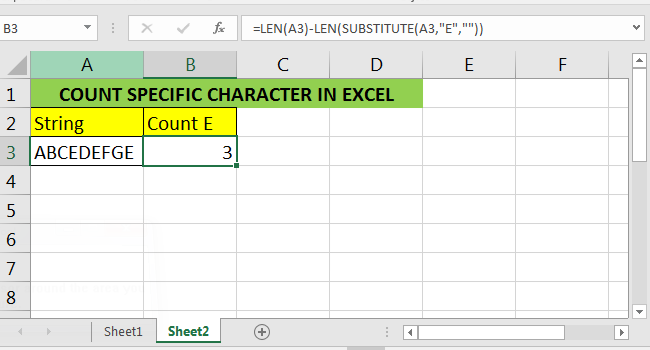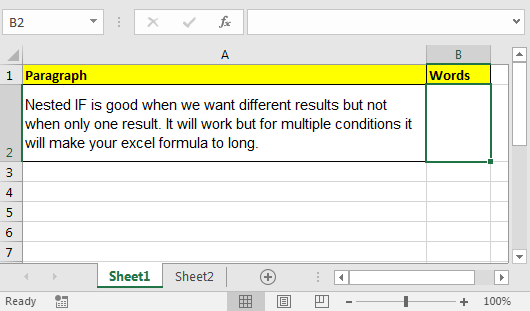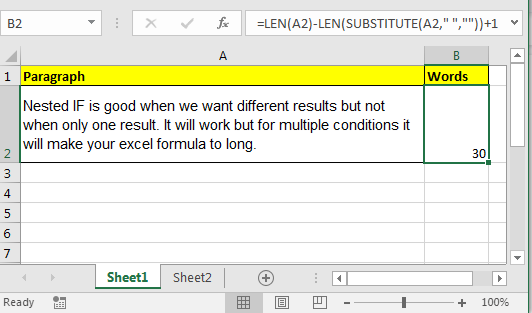# How to Count Characters in a Cell in Excel

If we can count the total number of characters in a cell using LEN, we can also count specific characters in a cell. We just need a helping hand. And that helping hand is SUBSTITUTE function of excel. Let's start counting.Generic Formula To Count Specific Characters in Excel - Case sensitive

=LEN(cell)-LEN(SUBSTITUTE(cell,"character/s",""))

Generic Formula To Count Specific Characters in a Cell - Case Insensitive

=LEN(cell)-LEN(SUBSTITUTE(UPPER(cell),"CHARACTER/S IN CAPITAL LETTER","'))

LEN Function returns total number of characters in a string or cell.

SUBSTITUTE function replaces the given character (or set of characters) with another given character (or set of characters). To eliminate character/s we use “” (blank) as a new character. Remember SUBSTITUTE is case sensitive.

Now let's see it in action.

### Example - Count Words in A Para

Here, in cell A2, I have a paragraph. Now I want to count words in it. To do so, we will count spaces in the sentence because:Total Number of Words = Total Number of Spaces +1

Write this formula in Cell B2

Formula To Count Words in A Paragraph

=LEN(A2)-LEN(SUBSTITUTE(A2," ","")) +1### How Does It work?

• LEN(A2): This part will return total number of characters in excel.
• LEN(SUBSTITUTE(A2," ","")
• SUBSTITUTE(A2," ",""): First, this part will replace all spaces with blanks. We will now have a string without spaces.
• LEN(SUBSTITUTE(A2," ",""): Now this part will give return length of this altered text.
• LEN(A2)-LEN(SUBSTITUTE(A2," ","")): This will return total number of spaces. Now this is equivalent to:
• number of characters with spaces - number of characters without spaces = total number of spaces.
• LEN(A2)-LEN(SUBSTITUTE(A2," ","")) +1 : Now this translates to:
• Number of spaces + 1 = total number of words in a paragraph.

So yeah guys, this is the way you can count any character in a string in excel. Just replace the character/s with blank (“”) and subtract its length from original text.

Related Articles:

How to Check If Cell Contains Specific Text in Excel

How to Check A list of Texts In String in Excel

How to COUNTIFS Two Criteria Match in Excel

How to COUNTIFS With OR For Multiple Criteria in Excel

Popular Articles:

50 Excel Shortcuts to Increase Your Productivity

How to use the VLOOKUP Function in Excel

How to use the COUNTIF function in Excel

How to use the SUMIF Function in Excel

Terms and Conditions of use

The applications/code on this site are distributed as is and without warranties or liability. In no event shall the owner of the copyrights, or the authors of the applications/code be liable for any loss of profit, any problems or any damage resulting from the use or evaluation of the applications/code.